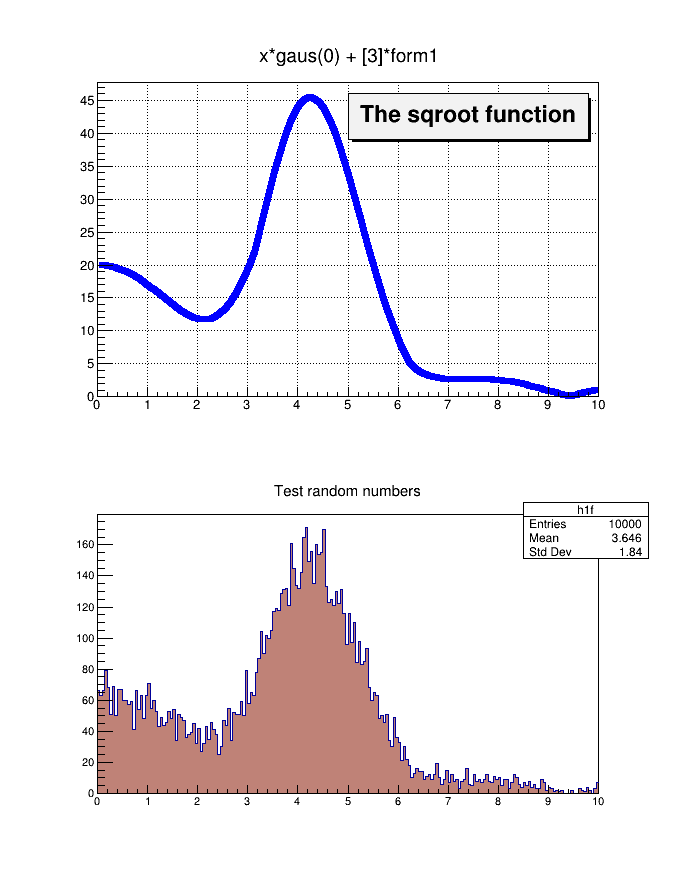# fillrandom¶

Fill a 1-D histogram from a parametric function. Original tutorial by Rene Brun.

Author: Alberto Ferro
This notebook tutorial was automatically generated with ROOTBOOK-izer from the macro found in the ROOT repository on Tuesday, August 16, 2022 at 09:36 AM.

In :
import ROOT

c1 = ROOT.TCanvas("c1","The FillRandom example",200,10,700,900)
ROOT.gBenchmark.Start("fillrandom")

form1 = ROOT.TFormula("form1","abs(sin(x)/x)")
sqroot = ROOT.TF1("sqroot","x*gaus(0) + *form1",0,10)
sqroot.SetParameters(10,4,1,20)
sqroot.SetLineColor(4)
sqroot.SetLineWidth(6)
sqroot.Draw()
lfunction = ROOT.TPaveLabel(5,39,9.8,46,"The sqroot function")
lfunction.Draw()
c1.Update()

h1f = ROOT.TH1F("h1f","Test random numbers",200,0,10)
h1f.SetFillColor(45)
h1f.FillRandom("sqroot",10000)
h1f.Draw()
c1.Update()

f = ROOT.TFile("fillrandom-py.root","RECREATE")
form1.Write()
sqroot.Write()
h1f.Write()
ROOT.gBenchmark.Show("fillrandom")

Welcome to JupyROOT 6.27/01


Draw all canvases

In :
from ROOT import gROOT
gROOT.GetListOfCanvases().Draw()